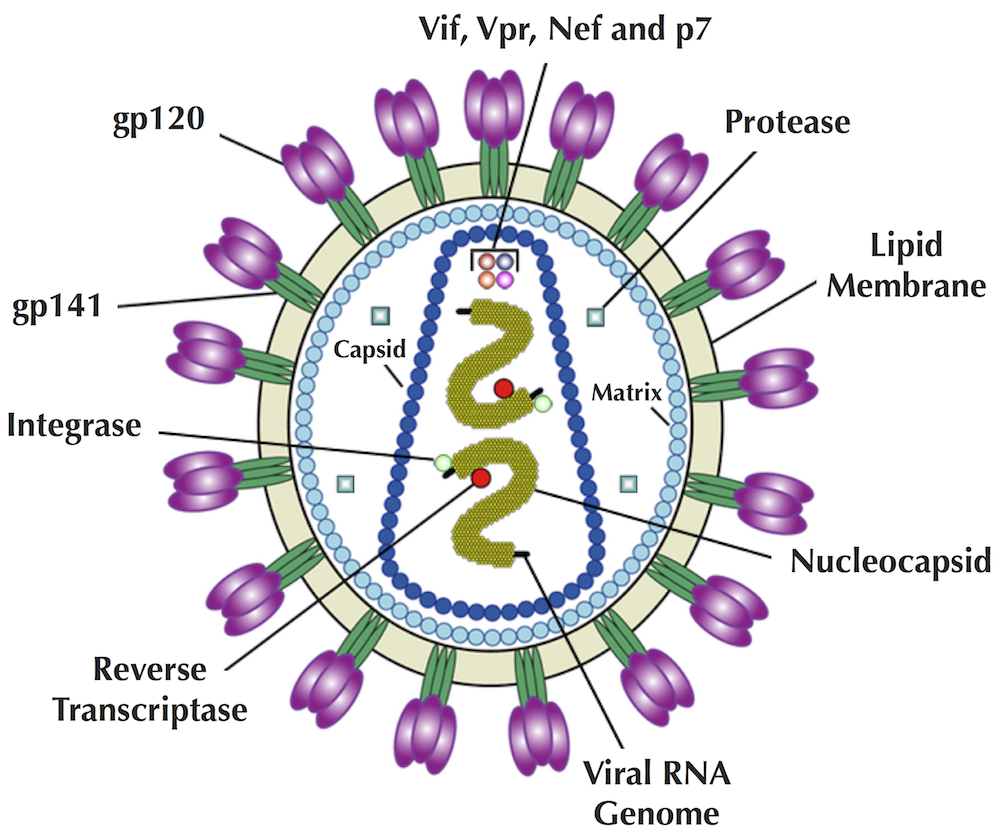Coursera Week 1

How did the Spike protein get its name?

Viruses form spherical particles, and their viral envelopes are studded with many little "spikes" formed by viral proteins. For example, HIV particles are embedded into the viral envelope with 72 spikes formed by gp120 and gp41 proteins (see figure below).How can I find the distance between two leaves in a tree?

There are a number of different ways to find the distance between nodes v and w, but if you don’t want to write a new program, you can use the solution to the Longest Path in a DAG problem from the chapter on sequence alignment. To do so, construct a directed graph by starting with v and orienting the edge incident to v so that it points away from v. Then, walk outward in the tree, adding all edges to the directed graph so that they are oriented away from v. When you encounter w, stop adding edges. Then simply find the length of a longest path connecting v to w.

Why does every tree with n nodes have exactly n − 1 edges?

Take an arbitrary leaf in a tree and delete it along with the edge incident to it. The number of nodes and edges in the resulting smaller tree have both been reduced by 1. Find a leaf in the resulting tree and remove it. The number of nodes and edges in the resulting (even smaller) tree has been reduced by 1 again. We iterate until we obtain a tree consisting of a single node (and no edges). Since we have removed the same number of nodes and edges during this iterative procedure, the number of nodes in the original tree exceeds the number of edges by 1.

Where should I add a new node to the tree T in AdditivePhylogeny?

To add a new node at distance x from leaf i on the path between i and leaf k, we need to first construct the path from i to k. If this path consists of edges e1, e2, … , et of lengths l1, … , lt, we will compute the length lengthi of the first i edges in this path as e1 + e2 + … + ei. As soon as lengthi exceeds x (i.e., lengthi-1 < x < lengthi), we know that the new node should be added at distance x - lengthi-1 from the start of the i-th edge. It is also possible to attach a new node at an existing node, which is the subject of the next question.

Is it possible for the tree produced by AdditivePhylogeny to have a node of degree larger than 3?

Yes. This will happen precisely when the attachment point of a leaf occurs at an existing internal node.

Coursera Week 2

Is it possible for NeighborJoining to produce a tree with a negative edge weight?

Yes. The neighbor-joining algorithm does sometimes assign negative edge weights when given a non-additive matrix. However, for some other non-additive matrices, it produces a tree whose weights are all non-negative (such as the matrix below, which is non-additive, but whose neighbor-joining tree has exclusively positive weights).

Coursera Week 3

How did 18S ribosomal RNA help resolve evolutionary controversies?

The first large-scale attempt to reconstruct the phylogeny of the entire animal kingdom was based on analyzing 18S RNA (see figure below). Before this work, there were many controversies with respect to the evolutionary relationships between distantly related animals because comparative anatomy and embryology approaches are not very effective for resolving deep branches in animal evolution.Figure: The first evolutionary tree of the animal kingdom, constructed from 18S RNA.